CBSE (Science) Class 11CBSE
Share

Books Shortlist
Your shortlist is empty

# NCERT solutions for Class 11 Physics chapter 5 - Laws of Motion

## Chapter 5 - Laws of Motion

#### Pages 109 - 113

Q 1.1 | Page 109

Give the magnitude and direction of the net force acting on a drop of rain falling down with a constant speed

Q 1.2 | Page 109

Give the magnitude and direction of the net force acting on a cork of mass 10 g floating on water,

Q 1.3 | Page 109

Give the magnitude and direction of the net force acting on a kite skillfully held stationary in the sky

Q 1.4 | Page 109

Give the magnitude and direction of the net force acting on a car moving with a constant velocity of 30 km/h on a rough road

Q 1.5 | Page 109

Give the magnitude and direction of the net force acting on a high-speed electron in space far from all material objects, and free of electric and magnetic fields.

Q 2 | Page 109

A pebble of mass 0.05 kg is thrown vertically upwards. Give the direction and magnitude of the net force on the pebble,

(a) during its upward motion,

(b) during its downward motion,

(c) at the highest point where it is momentarily at rest. Do your answers change if the pebble was thrown at an angle of 45° with the horizontal direction?

Ignore air resistance.

Q 3.1 | Page 109

Give the magnitude and direction of the net force acting on a stone of mass 0.1 kg,  just after it is dropped from the window of a stationary train.

Q 3.2 | Page 109

Give the magnitude and direction of the net force acting on a stone of mass 0.1 kg, just after it is dropped from the window of a train running at a constant velocity of 36 km/h,

Q 3.3 | Page 109

Give the magnitude and direction of the net force acting on a stone of mass 0.1 kg, just after it is dropped from the window of a train accelerating with 1 m s–2,

Q 3.4 | Page 110

Give the magnitude and direction of the net force acting on a stone of mass 0.1 kg, lying on the floor of a train which is accelerating with 1 m s–2, the stone being at rest relative to the train. Neglect air resistance throughout.

Q 4 | Page 110

One end of a string of length is connected to a particle of mass and the other to a small peg on a smooth horizontal table. If the particle moves in a circle with speed vthe net force on the particle (directed towards the centre) is

(i) T

(ii) T - (mv^2)/l

(iii) T + (mv^2)/l

(iv) 0

is the tension in the string. [Choose the correct alternative].

Q 5 | Page 110

A constant retarding force of 50 N is applied to a body of mass 20 kg moving initially with a speed of 15 ms–1. How long does the body take to stop?

Q 6 | Page 110

A constant force acting on a body of mass 3.0 kg changes its speed from 2.0 m s–1 to 3.5 m s–1 in 25 s. The direction of the motion of the body remains unchanged. What is the magnitude and direction of the force?

Q 7 | Page 110

A body of mass 5 kg is acted upon by two perpendicular forces 8 N and 6 N. Give the magnitude and direction of the acceleration of the body

Q 8 | Page 110

The driver of a three-wheeler moving with a speed of 36 km/h sees a child standing in the middle of the road and brings his vehicle to rest in 4.0 s just in time to save the child. What is the average retarding force on the vehicle? The mass of the three-wheeler is 400 kg and the mass of the driver is 65 kg.

Q 9 | Page 110

A rocket with a lift-off mass 20,000 kg is blasted upwards with an initial acceleration of 5.0 m s–2. Calculate the initial thrust (force) of the blast.

Q 10 | Page 110

A body of mass 0.40 kg moving initially with a constant speed of 10 m s–1 to the north is subject to a constant force of 8.0 N directed towards the south for 30 s. Take the instant the force is applied to be = 0, the position of the body at that time to be = 0, and predict its position at t = –5 s, 25 s, 100 s.

Q 11 | Page 110

A truck starts from rest and accelerates uniformly at 2.0 m s–2. At = 10 s, a stone is dropped by a person standing on the top of the truck (6 m high from the ground). What are the (a) velocity, and (b) acceleration of the stone at = 11 s? (Neglect air resistance.)

Q 12 | Page 110

A bob of mass 0.1 kg hung from the ceiling of a room by a string 2 m long is set into oscillation. The speed of the bob at its mean position is 1 m s–1. What is the trajectory of the bob if the string is cut when the bob is (a) at one of its extreme positions, (b) at its mean position

Q 13 | Page 110

A man of mass 70 kg stands on a weighing scale in a lift which is moving

(a) upwards with a uniform speed of 10 m s–1,

(b) downwards with a uniform acceleration of 5 m s–2,

(c) upwards with a uniform acceleration of 5 m s–2.

What would be the readings on the scale in each case?

(d) What would be the reading if the lift mechanism failed and it hurtled down freely under gravity?

Q 14 | Page 110

Figure shows the position-time graph of a particle of mass 4 kg. What is the (a) force on the particle for t < 0, t > 4 s< t < 4 s? (b) impulse at t = 0 and t = 4 s? (Consider one-dimensional motion only).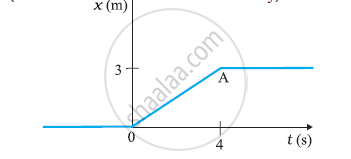Q 15 | Page 111

Two bodies of masses 10 kg and 20 kg respectively kept on a smooth, horizontal surface are tied to the ends of a light string. A horizontal force F = 600 N is applied to (i) A, (ii) B along the direction of string. What is the tension in the string in each case?

Q 16 | Page 111

Two masses 8 kg and 12 kg are connected at the two ends of a light inextensible string that goes over a frictionless pulley. Find the acceleration of the masses, and the tension in the string when the masses are released.

Q 17 | Page 111

A nucleus is at rest in the laboratory frame of reference. Show that if it disintegrates into two smaller nuclei the products must move in opposite directions.

Q 18 | Page 111

Two billiard balls, each of mass 0.05 kg, moving in opposite directions with speed 6 ms-1collide and rebound with the same speed. What is the impulse imparted to each ball due to the other?

Q 19 | Page 111

A shell of mass 0.020 kg is fired by a gun of mass 100 kg. If the muzzle speed of the shell is 80 m s–1, what is the recoil speed of the gun?

Q 20 | Page 111

A batsman deflects a ball by an angle of 45° without changing its initial speed which is equal to 54 km/h. What is the impulse imparted to the ball? (Mass of the ball is 0.15 kg.)

Q 21 | Page 111

A stone of mass 0.25 kg tied to the end of a string is whirled round in a circle of radius 1.5 m with a speed of 40 rev./min in a horizontal plane. What is the tension in the string? What is the maximum speed with which the stone can be whirled around if the string can withstand a maximum tension of 200 N?

Q 22 | Page 111

If, in Exercise 5.21, the speed of the stone is increased beyond the maximum permissible value, and the string breaks suddenly, which of the following correctly describes the trajectory of the stone after the string breaks:

(a) the stone moves radially outwards,

(b) the stone flies off tangentially from the instant the string breaks,

(c) the stone flies off at an angle with the tangent whose magnitude depends on the speed of the particle ?

Q 23.1 | Page 111

Explain why a horse cannot pull a cart and run in empty space

Q 23.2 | Page 111

Explain why passengers are thrown forward from their seats when a speeding bus stops suddenly

Q 23.3 | Page 111

Explain why it is easier to pull a lawn mower than to push it,

Q 23.4 | Page 111

Explain why  a cricketer moves his hands backwards while holding a catch.

Q 24 | Page 111

Figure shows the position-time graph of a body of mass 0.04 kg. Suggest a suitable physical context for this motion. What is the time between two consecutive impulses received by the body ? What is the magnitude of each impulse ?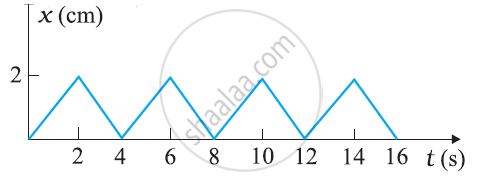Q 25 | Page 111

Figure  shows a man standing stationary with respect to a horizontal conveyor belt that is accelerating with 1 m s–2. What is the net force on the man? If the coefficient of static friction between the man’s shoes and the belt is 0.2, up to what acceleration of the belt can the man continue to be stationary relative to the belt? (Mass of the man = 65 kg.)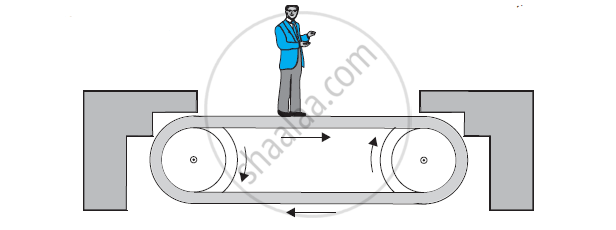Q 26 | Page 112

A stone of mass tied to the end of a string revolves in a vertical circle of radius R. The net forces at the lowest and highest points of the circle directed vertically downwards are: [Choose the correct alternative]

 Lowest Point Highest Point a) mg – T1 mg + T2 b) mg + T1 mg – T2 c) mg + T1 –(m_v_1^2)/R mg – T2 + (mv_1^2)/R d) mg – T1 – (mv)/R mg + T2 + (mv_1^2)/R

Tand v1 denote the tension and speed at the lowest point. Tand v2 denote corresponding values at the highest point.

Q 27 | Page 112

A helicopter of mass 1000 kg rises with a vertical acceleration of 15 m s–2. The crew and the passengers weigh 300 kg. Give the magnitude and direction of the

(a) force on the floor by the crew and passengers,

(b) action of the rotor of the helicopter on the surrounding air,

(c) force on the helicopter due to the surrounding air.

Q 28 | Page 113

A stream of water flowing horizontally with a speed of 15 m s–1 gushes out of a tube of cross-sectional area 10–2 m2, and hits a vertical wall nearby. What is the force exerted on the wall by the impact of water, assuming it does not rebound?

Q 29 | Page 112

Ten one-rupee coins are put on top of each other on a table. Each coin has a mass m. Give the magnitude and direction of

(a) the force on the 7th coin (counted from the bottom) due to all the coins on its top,

(b) the force on the 7th coin by the eighth coin,

(c) the reaction of the 6th coin on the 7th coin.

Q 30 | Page 112

An aircraft executes a horizontal loop at a speed of 720 km/h with its wings banked at 15°. What is the radius of the loop?

Q 31 | Page 112

A train runs along an unbanked circular track of radius 30 m at a speed of 54 km/h. The mass of the train is 106 kg. What provides the centripetal force required for this purpose – The engine or the rails? What is the angle of banking required to prevent wearing out of the rail

Q 32 | Page 113

A block of mass 25 kg is raised by a 50 kg man in two different ways as shown in Figure. What is the action on the floor by the man in the two cases? If the floor yields to a normal force of 700 N, which mode should the man adopt to lift the block without the floor yielding?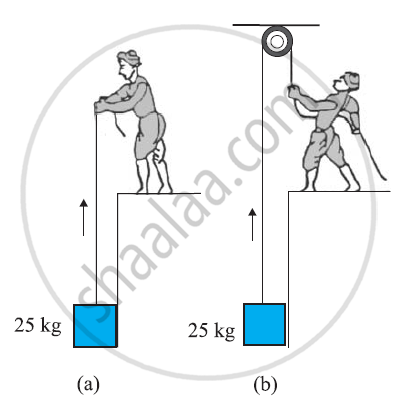Q 33 | Page 113

A monkey of mass 40 kg climbs on a rope in given Figure which can stand a maximum tension of 600 N. In which of the following cases will the rope break: the monkey

(a) climbs up with an acceleration of 6 m s–2

(b) climbs down with an acceleration of 4 m s–2

(c) climbs up with a uniform speed of 5 m s–1

(d) falls down the rope nearly freely under gravity?

(Ignore the mass of the rope).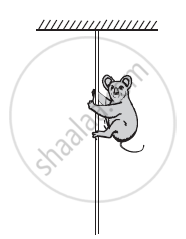Q 34 | Page 113

Two bodies and of masses 5 kg and 10 kg in contact with each other rest on a table against a rigid wall (Figure). The coefficient of friction between the bodies and the table is 0.15. A force of 200 N is applied horizontally to A. What are (a) the reaction of the partition (b) the action-reaction forces between and B?What happens when the wall is removed? Does the answer to (b) change, when the bodies are in motion? Ignore the difference between μs and μk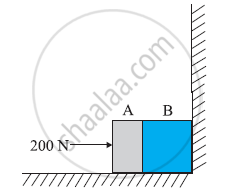Q 35 | Page 113

A block of mass 15 kg is placed on a long trolley. The coefficient of static friction between the block and the trolley is 0.18. The trolley accelerates from rest with 0.5 ms–2 for 20 s and then moves with uniform velocity. Discuss the motion of the block as viewed by (a) a stationary observer on the ground, (b) an observer moving with the trolley.

Q 36 | Page 113

The rear side of a truck is open and a box of 40 kg mass is placed 5 m away from the open end as shown in Figure. The coefficient of friction between the box and the surface below it is 0.15. On a straight road, the truck starts from rest and accelerates with 2 m s–2. At what distance from the starting point does the box fall off the truck? (Ignore the size of the box).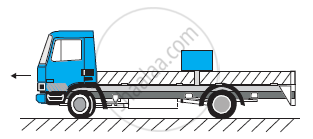Q 37 | Page 113

A disc revolves with a speed of 33 1/3 rev/min, and has a radius of 15 cm. Two coins are placed at 4 cm and 14 cm away from the centre of the record. If the co-efficient of friction between the coins and the record is 0.15, which of the coins will revolve with the record?

Q 38 | Page 113

You may have seen in a circus a motorcyclist driving in vertical loops inside a ‘death-well’ (a hollow spherical chamber with holes, so the spectators can watch from outside). Explain clearly why the motorcyclist does not drop down when he is at the uppermost point, with no support from below. What is the minimum speed required at the uppermost position to perform a vertical loop if the radius of the chamber is 25 m?

Q 39 | Page 113

A 70 kg man stands in contact against the inner wall of a hollow cylindrical drum of radius 3 m rotating about its vertical axis with 200 rev/min. The coefficient of friction between the wall and his clothing is 0.15. What is the minimum rotational speed of the cylinder to enable the man to remain stuck to the wall (without falling) when the floor is suddenly removed?

Q 40 | Page 113

A thin circular loop of radius rotates about its vertical diameter with an angular frequency ωShow that a small bead on the wire loop remains at its lowermost point for omega <= sqrt(g/R) .What is the angle made by the radius vector joining the centre to the bead with the vertical downward direction for omega = sqrt("2g"/R) ?Neglect friction.

## NCERT solutions for Class 11 Physics chapter 5 - Laws of Motion

NCERT solutions for Class 11 Physics chapter 5 (Laws of Motion) include all questions with solution and detail explanation. This will clear students doubts about any question and improve application skills while preparing for board exams. The detailed, step-by-step solutions will help you understand the concepts better and clear your confusions, if any. Shaalaa.com has the CBSE Physics Textbook for Class 11 Part 1 solutions in a manner that help students grasp basic concepts better and faster.

Further, we at shaalaa.com are providing such solutions so that students can prepare for written exams. NCERT textbook solutions can be a core help for self-study and acts as a perfect self-help guidance for students.

Concepts covered in Class 11 Physics chapter 5 Laws of Motion are Intuitive Concept of Force, Newton'S First Law of Motion, Newton’s Second Law of Motion, Newton's Third Law of Motion, Law of Conservation of Linear Momentum and Its Applications, Equilibrium of a Particle, Static and Kinetic Friction, Laws of Friction, Common Forces in Mechanics, Dynamics of Uniform Circular Motion - Centripetal Force, Examples of Circular Motion (Vehicle on a Level Circular Road, Vehicle on a Banked Road), Inertia, Lubrication - (Laws of Motion), Conservation of Momentum, Solving Problems in Mechanics, Aristotle’s Fallacy, The Law of Inertia, Circular Motion.

Using NCERT Class 11 solutions Laws of Motion exercise by students are an easy way to prepare for the exams, as they involve solutions arranged chapter-wise also page wise. The questions involved in NCERT Solutions are important questions that can be asked in the final exam. Maximum students of CBSE Class 11 prefer NCERT Textbook Solutions to score more in exam.

Get the free view of chapter 5 Laws of Motion Class 11 extra questions for Physics and can use shaalaa.com to keep it handy for your exam preparation

S